Function Repository Resource:

# IndependentComponentAnalysis

Decompose a matrix into Independent Component Analysis matrix factors

Contributed by: Anton Antonov
 ResourceFunction["IndependentComponentAnalysis"][mat,k] decomposes the matrix mat into k components. ResourceFunction["IndependentComponentAnalysis"][mat,k,opts] decomposes mat using the options opts.

## Details and Options

The Independent Component Analysis (ICA) is a matrix factorization method that utilizes Gram-Schmidt orthogonalization by considering two vectors (two signals) to be orthogonal if their difference is Gaussian white noise.
The argument mat can be a square or rectangular matrix.
The columns of mat are interpreted as signals.
ResourceFunction["IndependentComponentAnalysis"] accepts the following options:
 "InitialUnmixingMatrix" Automatic initial un-mixing matrix "NegEntropyFactor" 1 negative entropy factor "NonGaussianityFunction" Automatic non-Gaussianity measure function "RowNorm" False whether to normalize the matrix rows at each step MaxSteps 200 maximum steps of the ICA interation process PrecisionGoal 6 precision goal
For some data, at least several executions are required in order to get components that provide satisfactory interpretation.
The negative entropy factor is specified with the option "NegEntropyFactor". The negative entropy factor controls how aggressively ICA learns from the "landscape" produced by the non-Gaussianity measure function (specified with "NonGaussianityFunction").
The function ResourceFunction["IndependentComponentAnalysis"] uses SingularValueDecomposition internally.

## Examples

### Basic Examples (3)

Here is a random integer matrix:

 In:=Out=Here are the Independent Component Analysis matrix factors:

 In:=Out=Here is the matrix product of the obtained factors:

 In:=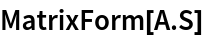Out=### Scope (6)

Here is a random matrix with its first two columns having much larger magnitudes than the rest:

 In:=Plot the values:

 In:=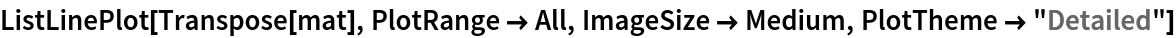Out=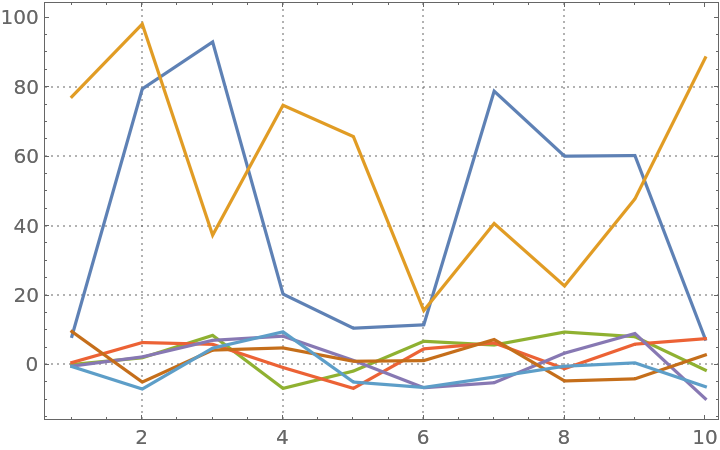Compute the ICA matrix factors:

 In:=Here is the relative error of the approximation by the obtained matrix factorization:

 In:=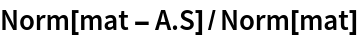Out=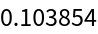Here is the relative error for the first three columns:

 In:=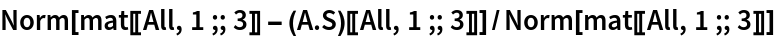Out=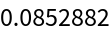Here are comparison plots:

 In:=Out=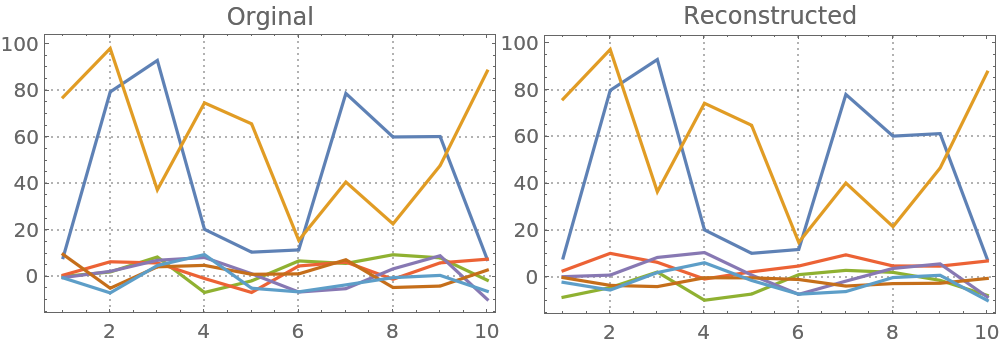### Options (4)

#### InitialUnmixingMatrix (2)

Using the option "InitialUnmixingMatrix", we can influence the results by providing initial un-mixing matrix for ICA's iteration process. Here we compute ICA with different initial un-mixing matrices:

 In:=Plot the results:

 In:=Out=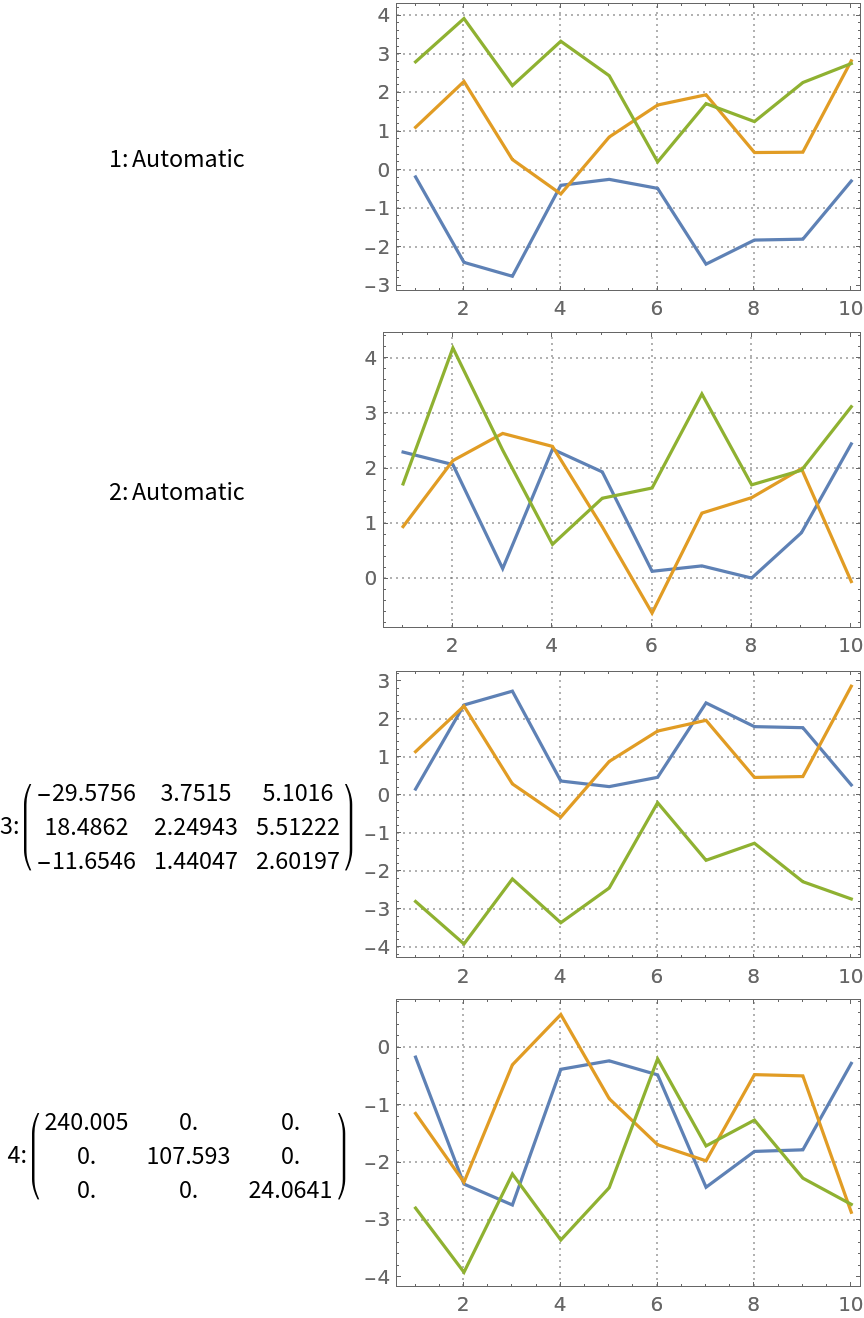#### NonGaussianityFunction (2)

ICA is essentially a Gram-Schmidt process that considers two signals to be orthogonal if their difference is Gaussian noise.

Here we compute ICA with different non-Gaussianity measure functions:

 In:=Plot the results:

 In:=Out=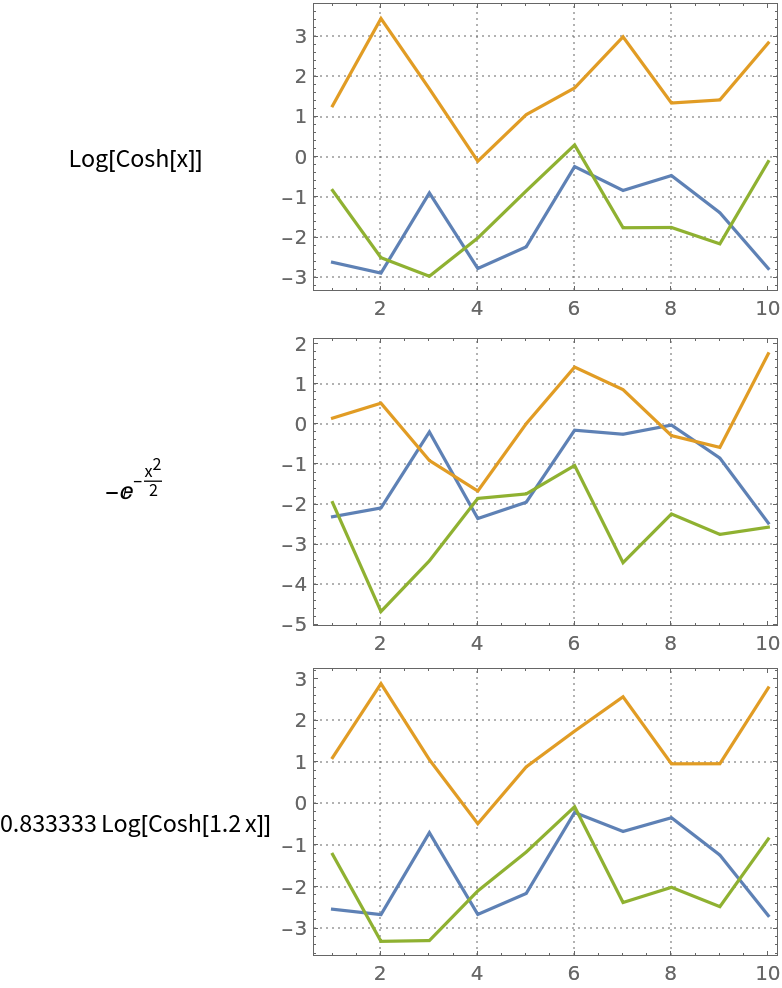Note that we used a small number of steps in order to obtain similar but different results. Using a sufficiently large number of steps (say, 20) we get almost the same results (with the matrix mat).

### Applications (8)

#### Blind signal separation (8)

Here are signal generating functions:

 In:=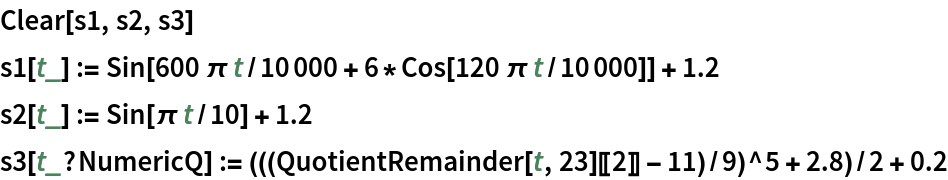Here is a mixing matrix:

 In:=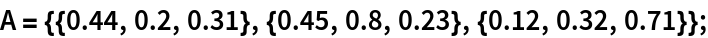Form a matrix of the signals:

 In:=Out=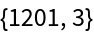Plot the values:

 In:=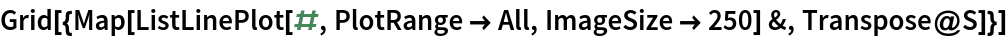Out=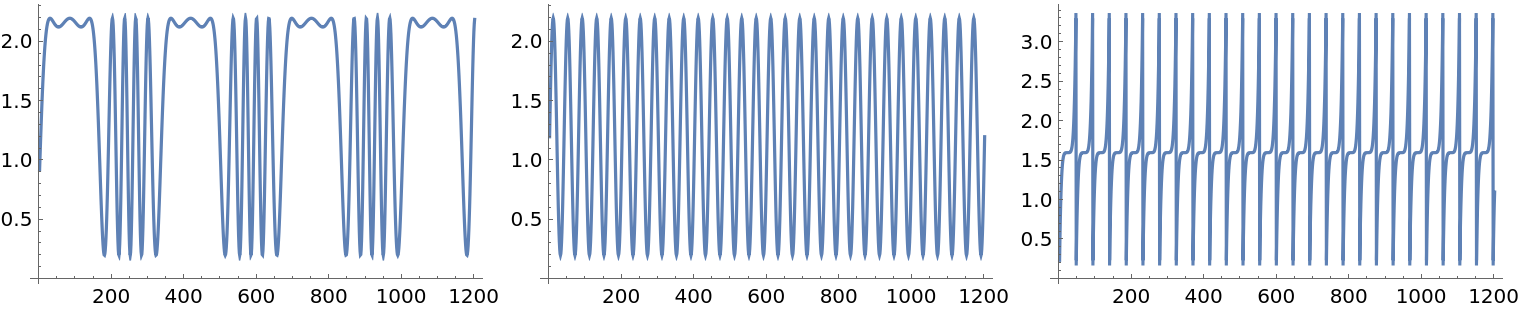Create and plot the mixed signals matrix:

 In:=Out=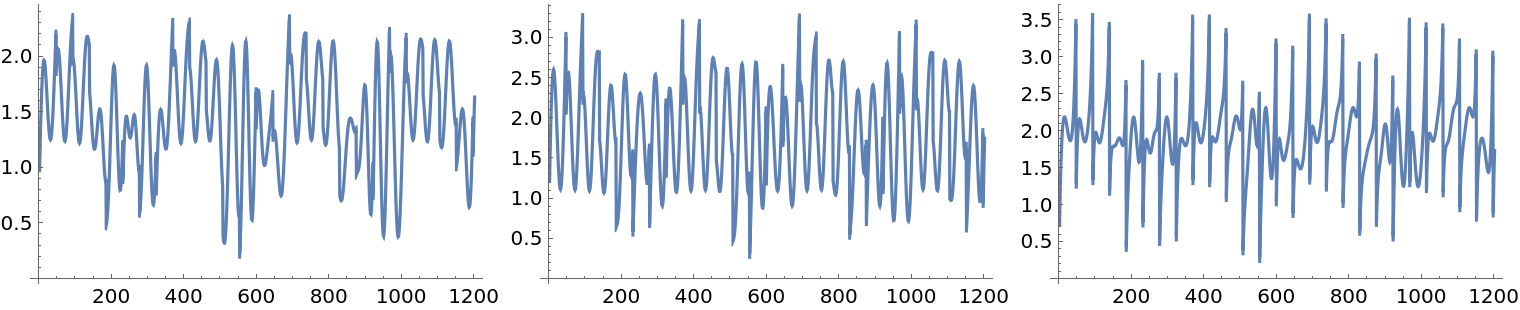Find the ICA decomposition:

 In:=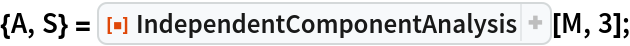Approximation norm:

 In:=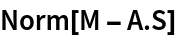Out=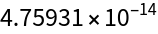Visualize the ICA-identified source signals:

 In:=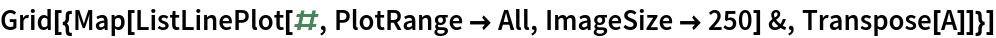Out=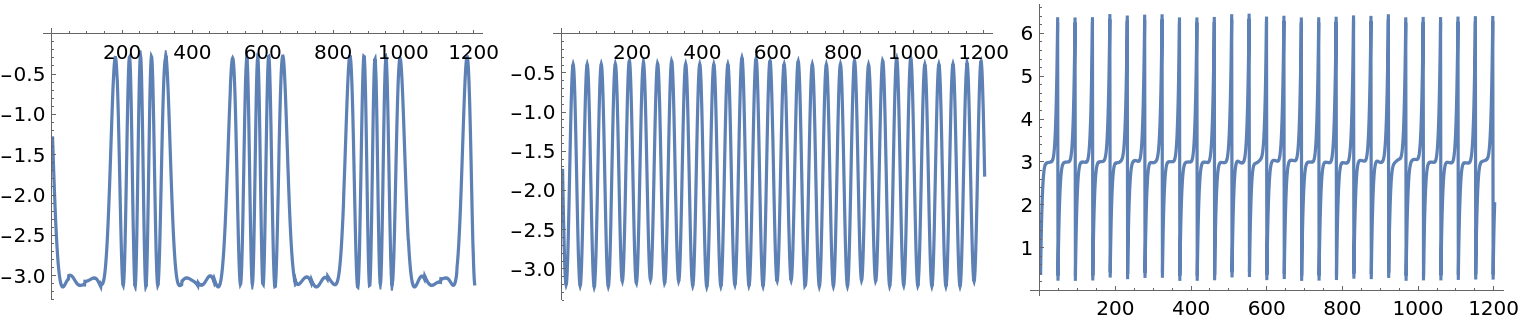### Possible Issues (3)

#### Outliers presence sensitivity (3)

The ICA computations can be sensitive to the presence of outliers. Here we add a small number of outliers to the original mixed signals:

 In:=Out=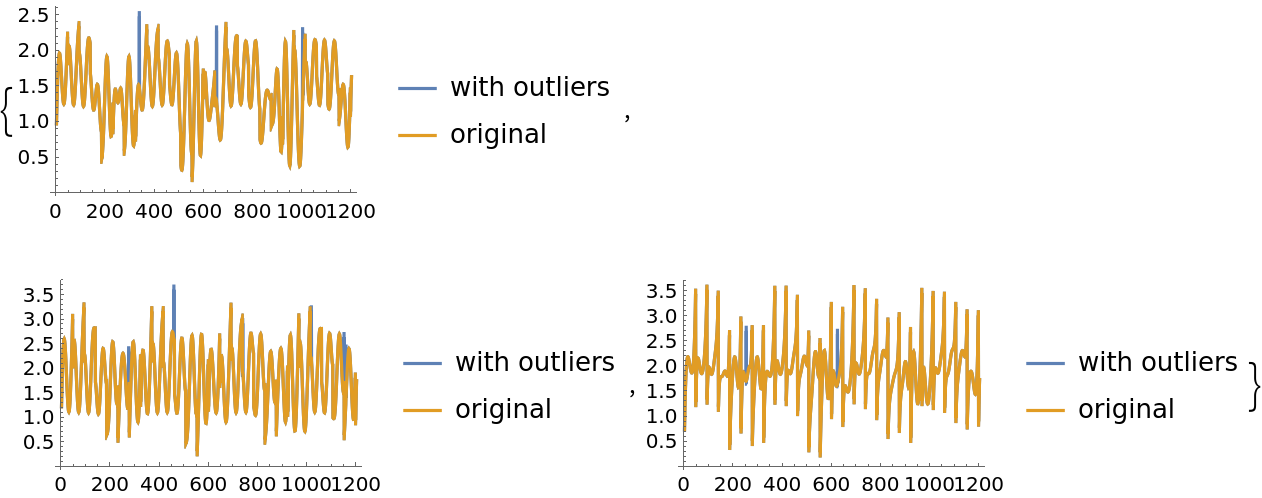Here we apply ICA to the new mixed signals:

 In:=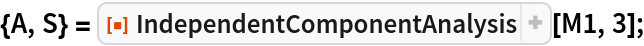We see that the found components are not that good compared to the components found for the original mixed signals data:

 In:=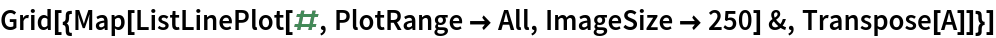Out=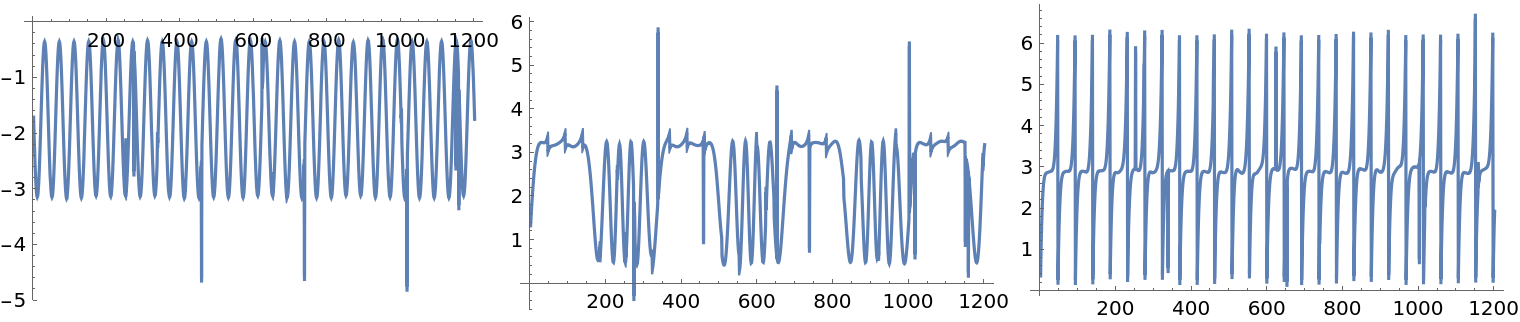Anton Antonov

## Version History

• 1.0.0 – 18 November 2019